# NCERT SOLUTION FOR CLASS 8 MATHS CHAPTER 6 SQUARES AND SQUARE ROOTS

The National Council of Educational Research and Training (NCERT) is an autonomous body of the Indian government that formulates the curricula for schools in India that are governed by the Central Board of Secondary Education (CBSE) and certain state boards. Therefore, students who will be taking the Class 10 tests administered by various boards should consult this NCERT Syllabus in order to prepare for those examinations, which in turn will assist those students get a passing score.

You can also download the NCERT SOLUTION FOR CLASS 8 MATHS CHAPTER 6 SQUARES AND SQUARE ROOTS to assist you in comprehensively reviewing the course material and achieving a higher score on your upcoming assessments.

Students are able to create a strong conceptual basis with the help of the NCERT Solutions PDFs available at SWC. This base plays a key part in the students’ preparation for the latter phases of the competitive examinations. We give comprehensive answers to challenges posed by NCERT in accordance with the methodology provided by CBSE. The students are able to effortlessly prepare all of the ideas that are presented in their respective courses better and more successfully, and they are even able to succeed in passing the most difficult competitive examinations, such as JEE Main, JEE Advanced, NEET, AIMS, and so on.

Your academic journey begins a new stage as you reach the eighth grade, and a new facet of learning awaits you. This is the year where pupils begin to acquire an interest in the topic at hand, and it is also the year in which you choose whether you will study business, humanity, or science in the following year (class 12). As a consequence of this, having a solid foundation of knowledge in fields such as mathematics and physics comes highly recommended. The NCERT textbook for class 8 is the greatest book now available on the market for enhancing one’s education in the areas of mathematics and science. Read up on the theory that is presented in the book for grade 8 and answer all of the questions that are posed in the practice section of the NCERT textbook.

## NCERT SOLUTION FOR CLASS 8 MATHS CHAPTER 6 SQUARES AND SQUARE ROOTS – Exercises

### NCERT Solutions for Class 8 Maths Exercise 6.1

Question 1.

What will be the unit digit of the squares of the following numbers:
(i) 81
(ii) 272
(iii) 799
(iv) 3853
(v) 1234
(vi) 26387
(vii) 52698
(viii) 99880
(ix) 12796
(x) 55555

Solution :
(i) The number 81 contains its unit’s place digit 1. So, square of 1 is 1.
Hence, unit’s digit of square of 81 is 1.
(ii) The number 272 contains its unit’s place digit 2. So, square of 2 is 4.
Hence, unit’s digit of square of 272 is 4.
(iii) The number 799 contains its unit’s place digit 9. So, square of 9 is 81.
Hence, unit’s digit of square of 799 is 1.
(iv) The number 3853 contains its unit’s place digit 3. So, square of 3 is 9.
Hence, unit’s digit of square of 3853 is 9.
(v) The number 1234 contains its unit’s place digit 4. So, square of 4 is 16.
Hence, unit’s digit of square of 1234 is 6.
(vi) The number 26387 contains its unit’s place digit 7. So, square of 7 is 49.
Hence, unit’s digit of square of 26387 is 9.
(vii) The number 52698 contains its unit’s place digit 8. So, square of 8 is 64.
Hence, unit’s digit of square of 52698 is 4.
(viii) The number 99880 contains its unit’s place digit 0. So, square of 0 is 0.
Hence, unit’s digit of square of 99880 is 0.
(ix) The number 12796 contains its unit’s place digit 6. So, square of 6 is 36.
Hence, unit’s digit of square of 12796 is 6.
(x) The number 55555 contains its unit’s place digit 5. So, square of 5 is 25.
Hence, unit’s digit of square of 55555 is 5.

Question 2.
The following numbers are obviously not perfect squares. Give reasons.
(i) 1057
(ii) 23453
(iii) 7928
(iv) 222222
(v) 64000
(vi) 89722
(vii) 222000
(viii) 505050

Solution :
(i) Since, perfect square numbers contain their unit’s place digit 1, 4, 5, 6, 9 and even numbers of 0.
Therefore 1057 is not a perfect square because its unit’s place digit is 7.
(ii) Since, perfect square numbers contain their unit’s place digit 0, 1, 4, 5, 6, 9 and even number of 0. Therefore 23453 is not a perfect square because its unit’s place digit is 3.
(iii) Since, perfect square numbers contain their unit’s place digit 0, 1, 4, 5, 6, 9 and even number of 0. Therefore 7928 is not a perfect square because its unit’s place digit is 8.
(iv) Since, perfect square numbers contain their unit’s place digit 0, 1, 4, 5, 6, 9 and even number of 0. Therefore 222222 is not a perfect square because its unit’s place digit is 2.
(v) Since, perfect square numbers contain their unit’s place digit 0, 1, 4, 5, 6, 9 and even number of 0. Therefore 64000 is not a perfect square because its unit’s place digit is single 0.
(vi) Since, perfect square numbers contain their unit’s place digit 0, 1, 4, 5, 6, 9 and even number of 0. Therefore 89722 is not a perfect square because its unit’s place digit is 2.
(vii) Since, perfect square numbers contain their unit’s place digit 0, 1, 4, 5, 6, 9 and even number of 0. Therefore 222000 is not a perfect square because its unit’s place digit is triple 0.

Question 3.
The squares of which of the following would be odd number:
(i) 431
(ii) 2826
(iii) 7779
(iv) 82004

Solution :
(i) 431 – Unit’s digit of given number is 1 and square of 1 is 1. Therefore, square of 431 would be an odd number.
(ii) 2826 – Unit’s digit of given number is 6 and square of 6 is 36. Therefore, square of 2826 would not be an odd number.
(iii) 7779 – Unit’s digit of given number is 9 and square of 9 is 81. Therefore, square of 7779 would be an odd number.
(iv) 82004 – Unit’s digit of given number is 4 and square of 4 is 16. Therefore, square of 82004 would not be an odd number.

Question 4.
Observe the following pattern and find the missing digits:
11² = 121
101²= 10201
1001²= 1002001
10001²= 1…….2…….1
100001²= 1……………………

Solution :
11²= 121
101²= 10201
1001²= 1002001
10001²= 10000200001
10000001²= 100000020000001

Question 5.

Observe the following pattern and supply the missing numbers:
11²= 121
101²= 10201
10101²= 102030201
1010101²= ………………………
10101011²= 10203040504030201

Solution :
11² = 121
101² = 10201
10101² = 102030201
1010101² = 1020304030201
10101010²= 10203040504030201

Question 6.
Using the given pattern, find the missing numbers:

1²  + 2²  + 2² = 3²

2²  + 3²  + 6² = 7²

3²  + 4²  + 12² = 13²

4²  + 5²  + _² = 21²

5²  + _²  + 30²  =  31²

6²  + _²  + _² = 7²

Solution :

1²  + 2²  + 2² = 3²

2²  + 3²  + 6² = 7²

3²  + 4²  + 12² = 13²

4²  + 5²  + 20² = 21²

5²  + _²  + 30²  =  31²

6²  + 7²  + 42² = 7²

Question 7.
(i) 1 + 3 + 5 + 7 + 9
(ii) 1 + 3 + 5 + 7 + 9 + 11 + 13 + 15 + 17 + 19
(iii) 1 + 3 + 5 + 7 + 9 + 11 + 13 + 15 + 17 + 19 + 21 + 23
Solution :
(i) Here, there are five odd numbers. Therefore square of 5 is 25.
∴1 + 3 + 5 + 7 + 9 = 52= 25
(ii) Here, there are ten odd numbers. Therefore square of 10 is 100.
∴ 1 + 3 + 5 + 7 + 9 + 11 + 13 + 15 + 17 + 19 = 102 = 100
(iii) Here, there are twelve odd numbers. Therefore square of 12 is 144.
1 + 3 + 5 + 7 + 9 + 11 + 13 + 15 + 17 + 19 + 21 + 23 = 122 = 144

Question 8.
(i) Express 49 as the sum of 7 odd numbers.
(ii) Express 121 as the sum of 11 odd numbers.

Solution :
(i) 49 is the square of 7. Therefore it is the sum of 7 odd numbers.
49 = 1 + 3 + 5 + 7 + 9 + 11 + 13
(ii) 121 is the square of 11. Therefore it is the sum of 11 odd numbers
121 = 1 + 3 + 5 + 7 + 9 + 11 + 13 + 15 + 17 + 19 + 21

Question 9.
How many numbers lie between squares of the following numbers:
(i) 12 and 13
(ii) 25 and 26
(iii) 99 and 100

Solution :
(i) Since, non-perfect square numbers between  and  (n + 1)² vare 2n

Here,  n= 12

Therefore, non-perfect square numbers between 12 and 13 =n2 =(n + 1)² = 24
(ii) Since, non-perfect square numbers between and are
Here, n= 25
Therefore, non-perfect square numbers between 25 and 26 =2n =2× 12= 50
(iii) Since, non-perfect square numbers between  and (n + 1)²  are  2n
Here, n = 99
Therefore, non-perfect square numbers between 99 and 100 = 2n = 2×99 = 198

### NCERT Solutions for Class 8 Maths Exercise 6.2

Question 1.
Find the squares of the following numbers:
(i) 32
(ii) 35
(iii) 86
(iv) 93
(v) 71
(vi) 46

Solution :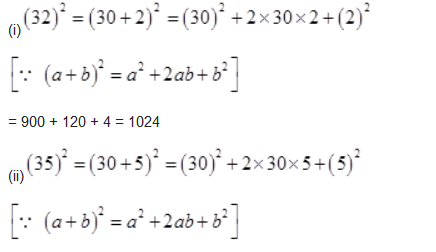= 900 + 300 + 25 = 1225

= 4900 + 140 + 1 = 5041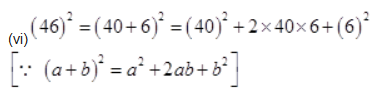= 1600 + 480 + 36 = 2116

Question 2.

Write a Pythagoras triplet whose one member is:
(i) 6
(ii) 14
(iii) 16
(iv) 18
Solution :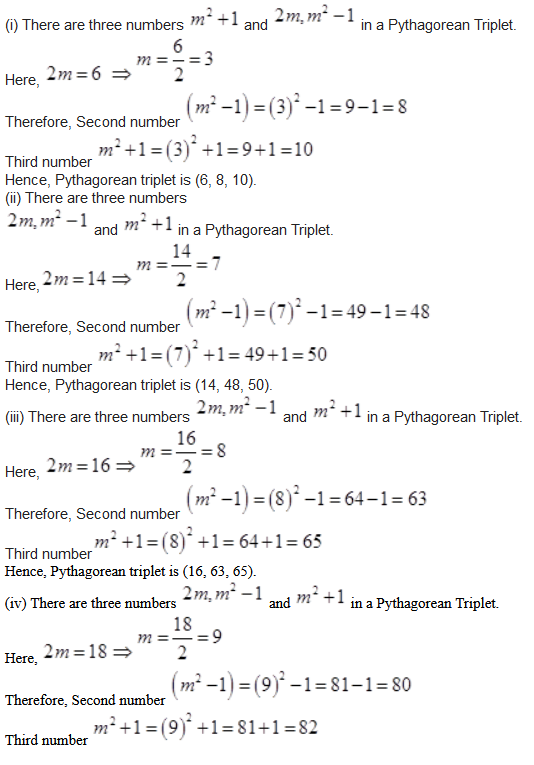### NCERT Solutions for Class 8 Maths Exercise 6.3

Question 1.

What could be the possible ‘one’s’ digits of the square root of each of the following numbers:
(i) 9801
(ii) 99856
(iii) 998001
(iv) 657666025

Solution :
Since, Unit’s digits of square of numbers are 0, 1, 4, 5, 6 and 9. Therefore, the possible unit’s digits of the given numbers are:
(i) 1 (ii) 6 (iii) 1 (iv) 5

Question 2.
Without doing any calculation, find the numbers which are surely not perfect squares:
(i) 153
(ii) 257
(iii) 408
(iv) 441
Solution :
Since, all perfect square numbers contain their unit’s place digits 0, 1, 4, 5, 6 and 9.
(i) But given number 153 has its unit digit 3. So it is not a perfect square number.
(ii) Given number 257 has its unit digit 7. So it is not a perfect square number.
(iii) Given number 408 has its unit digit 8. So it is not a perfect square number.
(iv) Given number 441 has its unit digit 1. So it would be a perfect square number

Question 3.
Find the square roots of 100 and 169 by the method of repeated subtraction.

Solution :
By successive subtracting odd natural numbers from 100,
100 – 1 = 99
99 – 3 = 96
96 – 5 = 91
91 – 7 = 84
84 – 9 = 75
75 – 11 = 64
64 – 13 = 51
51 – 15 = 36
36 – 17 = 19
19 – 19 = 0
This successive subtraction is completed in 10 steps.
Therefore √100 =10
By successive subtracting odd natural numbers from 169,
169 – 1 = 168
168 – 3 = 165
165 – 5 = 160
160 – 7 = 153
153 – 9 = 144
144 – 11 = 133
133 – 13 = 120
120 – 15 = 105
105 – 17 = 88
88 – 19 = 69
69 – 21 = 48
48 – 23 = 25
25 – 25 = 0
This successive subtraction is completed in 13 steps.
Therefore  √169 =13

Question 4.
Find the square roots of the following numbers by the Prime Factorization method:
(i) 729
(ii) 400
(iii) 1764
(iv) 4096
(v) 7744
(vi) 9604
(vii) 5929
(viii) 9216
(ix) 529
(x) 8100

Solution :
(i) 729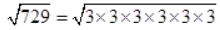=3x3x3
= 27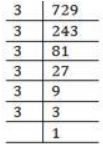(ii) 400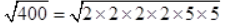(iii) 1764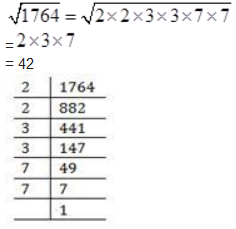(iv) 4096

= 64

(v) 7744

= 88

(vi) 9604

= 98

(vii) 5929

(viii) 9216

= 96

(ix) 529

= 23

(x) 8100

= 90

Question 5. For each of the following numbers, find the smallest whole number by which it should be multiplied so as to get a perfect square number. Also, find the square root of the square number so obtained:

(i) 252 (ii) 180

(iii) 1008 (iv) 2028

(v) 1458 (vi) 768

Solution :

(i) 252 =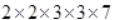Here, prime factor 7 has no pair. Therefore 252 must be multiplied by 7 to make it a perfect square.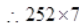= 1764

And (i)= 2×3×7= 42

(ii) 180 = 2×2×3×3×5

Here, prime factor 5 has no pair. Therefore 180 must be multiplied by 5 to make it a perfect square.

∴180 x 5 = 900

And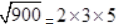= 30

(iii) 1008 =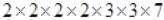Here, prime factor 7 has no pair. Therefore 1008 must be multiplied by 7 to make it a perfect square.

∴ 1008 × 7 = 7056

And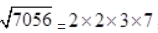= 84

(iv) 2028 =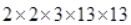Here, prime factor 3 has no pair. Therefore 2028 must be multiplied by 3 to make it a perfect square.

∴ 2028 × 3= 6084

And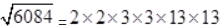= 78

(v) 1458 =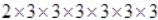Here, prime factor 2 has no pair. Therefore 1458 must be multiplied by 2 to make it a perfect square.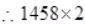= 2916

And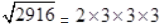= 54

(vi) 768 =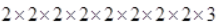Here, prime factor 3 has no pair. Therefore 768 must be multiplied by 3 to make it a perfect square.

∴ 768 × 3= 2304

And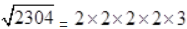= 48

### NCERT Solutions for Class 8 Maths Exercise 6.4

Question 1.
Find the square roots of each of the following numbers by Division method:
(i) 2304 (ii) 4489
(iii) 3481 (iv) 529
(v) 3249 (vi) 1369
(vii) 5776 (viii) 7921
(ix) 576 (x) 1024
(xi) 3136 (xii) 900

Solution :
(i) 2304
Hence, the square root of 2304 is 48.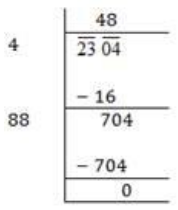(ii) 4489

Hence, the square root of 4489 is 67.

(iii) 3481

Hence, the square root of 3481 is 59.

(iv) 529

Hence, the square root of 529 is 23.

(v) 3249

Hence, the square root of 3249 is 57.

(vi) 1369

Hence, the square root of 1369 is 37.

(vii) 5776

Hence, the square root of 5776 is 76.

(viii) 7921

Hence, the square root of 7921 is 89.

(ix) 576

Hence, the square root of 576 is 24.

(x) 1024

Hence, the square root of 1024 is 32.

(xi) 3136

Hence, the square root of 3136 is 56.

(xii) 900

Hence, the square root of 900 is 30.

Question 2.

Find the number of digits in the square root of each of the following numbers (without any calculation):

(i) 64

(ii) 144

(iii) 4489

(iv) 27225

(v) 390625

Solution :

(i) Here, 64 contains two digits which is even.

Therefore, number of digits in square root =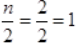(ii) Here, 144 contains three digits which is odd.

Therefore, number of digits in square root =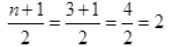(iii) Here, 4489 contains four digits which is even.

Therefore, number of digits in square root =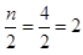(iv) Here, 27225 contains fivr digits which is odd.

Therefore, number of digits in square root =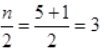(v) Here, 390625 contains six digits which is even.

Therefore, number of digits in square root =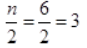Question 3.

Find the square root of the following decimal numbers:

(i) 2.56

(ii) 7.29

(iii) 51.84

(iv) 42.25

(v) 31.36

Solution :

(i) 2.56

Hence, the square root of 2.56 is 1.6.

(ii) 7.29

Hence, the square root of 7.29 is 2.7.

(iii) 51.84

Hence, the square root of 51.84 is 7.2.

(iv) 42.25

Hence, the square root of 42.25 is 6.5.

(v) 31.36

Hence, the square root of 31.36 is 5.6.

Question 4.

Find the least number which must be subtracted from each of the following numbers so as to get a perfect square. Also, find the square root of the perfect square so obtained:

(i) 402

(ii) 1989

(iii) 3250

(iv) 825

(v) 4000

Solution :

(i) 402

We know that, if we subtract the remainder from the number, we get a perfect square.

Here, we get remainder 2. Therefore 2 must be subtracted from 402 to get a perfect square.

∴ 402 – 2 = 400

Hence, the square root of 400 is 20.

(ii) 1989

We know that, if we subtract the remainder from the number, we get a perfect square.

Here, we get remainder 53. Therefore 53must be subtracted from 1989 to get a perfect square.

∴ 1989 – 53 = 1936

Hence, the square root of 1936 is 44.

(iii) 3250

We know that, if we subtract the remainder from the number, we get a perfect square.

Here, we get remainder 1. Therefore 1 must be subtracted from 3250 to get a perfect square.

∴3250 – 1 = 3249

Hence, the square root of 3249 is 57.

(iv) 825

We know that, if we subtract the remainder from the number, we get a perfect square.

Here, we get remainder 41. Therefore 41 must be subtracted from 825 to get a perfect square.

∴ 825 – 41 = 784

Hence, the square root of 784 is 28.

(v) 4000

We know that, if we subtract the remainder from the number, we get a perfect square.

Here, we get remainder 31. Therefore 31 must be subtracted from 4000 to get a perfect square.

∴ 4000 – 31 = 3969

Hence, the square root of 3969 is 63.

Question 5.

Find the least number which must be added to each of the following numbers so as to get a perfect square. Also, find the square root of the perfect square so obtained:

(i) 525

(ii) 1750

(iii) 252

(iv) 1825

(v) 6412

Solution :

(i) 525

Since remainder is 41.

Therefore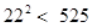Next perfect square number 23²= 529

= 529 – 525 = 4

∴ 525 + 4 = 529

Hence, the square root of 529 is 23.

(ii) 1750

Since remainder is 69.

Therefore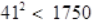Next perfect square number  42² = 1764

= 1764 – 1750 = 14

∴ 1750 + 14 = 1764

Hence, the square root of 1764 is 42.

(iii) 252

Since remainder is 27.

Therefore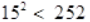Next perfect square number 16² = 256

= 256 – 252 = 4

∴ 252 + 4 = 256

Hence, the square root of 256 is 16.

(iv) 1825

Since remainder is 61.

Therefore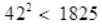Next perfect square number  43² = 1849

Hence, number to be added = 1849 – 1825 = 24

∴ 1825 + 24 = 1849

Hence, the square root of 1849 is 43.

(v) 6412

Since remainder is 12.

Therefore  80²  <  6412

Next perfect square number 81²= 6561

= 6561 – 6412 = 149

∴ 6412 + 149 = 6561

Hence, the square root of 6561 is 81.

Question 6.

Find the length of the side of a square whose area is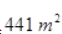?

Solution :

Let the length of side of a square be x meter.

Area of square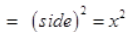According to question,

Hence, the length of side of a square is 21 m.

Question 7.

In a right triangle ABC,∠B = 90º

(i) If AB = 6 cm, BC = 8 cm, find AC.

(ii) If AC = 13 cm, BC = 5 cm, find AB.

Solution :

(a) Using Pythagoras theorem,

(b) Using Pythagoras theorem,

Question 8.

A gardener has 1000 plants. He wants to plant these in such a way that the number of rows and number of columns remain same. Find the minimum number of plants he needs more for this.

Solution :

Here, plants = 1000

Since remainder is 39.

Therefore  31² < 1000

Next perfect square number 32² = 1024

= 1024 – 1000 = 24

∴ 1000 + 24 = 1024

Hence, the gardener required 24 more plants.

Question 9.

There are 500 children in a school. For a P.T. drill they have to stand in such a manner that the number of rows is equal to number of columns. How many children would be left out in this arrangement?

Solution :

Here, Number of children = 500

By getting the square root of this number, we get,

In each row, the number of children is 22.

And left out children are 16.

## Conclusions for NCERT Solutions for Class 8 Maths Chapter 6

The academic team of SWC has provided NCERT answers for class 8 mathematics chapter-6. We’ve given solutions for all of Chapter 6’s exercises. These are step-by-step answers to all of the problems in Chapter 6 of the NCERT textbook. Read the first theoretical chapter. Make sure you’ve gone through the theoretical section of chapter 6 in the NCERT textbook and that you know the formula for the chapter.

When working through the exercises in the NCERT textbook, if you run into any type of difficulty or uncertainty, you may use the SWC NCERT Solutions for class 8 as a point of reference. While you are reading the theory from the textbook, it is imperative that you always have notes prepared. You should make an effort to understand things from the very beginning so that you may create a solid foundation in the topic. Use the NCERT as your parent book to ensure that you have a strong foundation. After you have finished reading the theoretical section of the textbook, you should go to additional reference books.